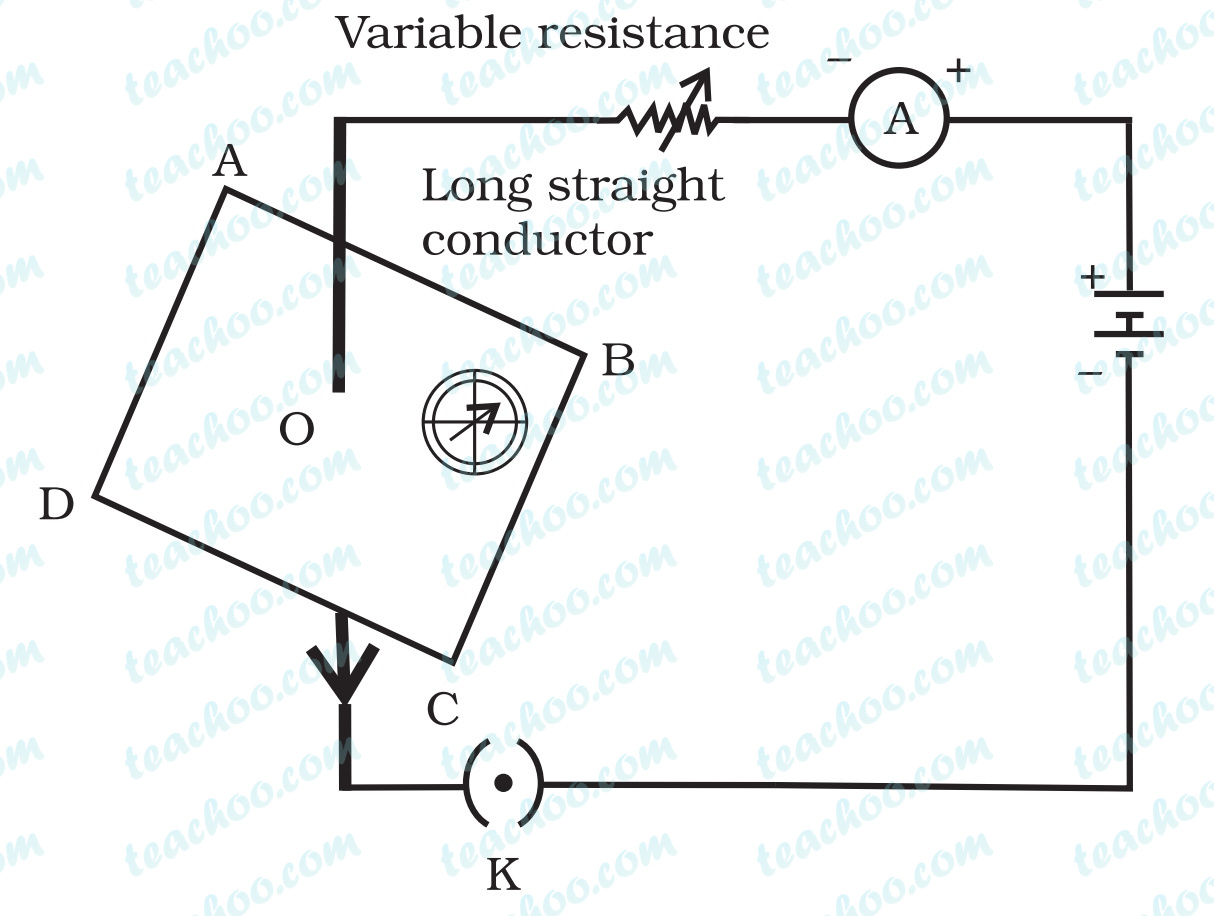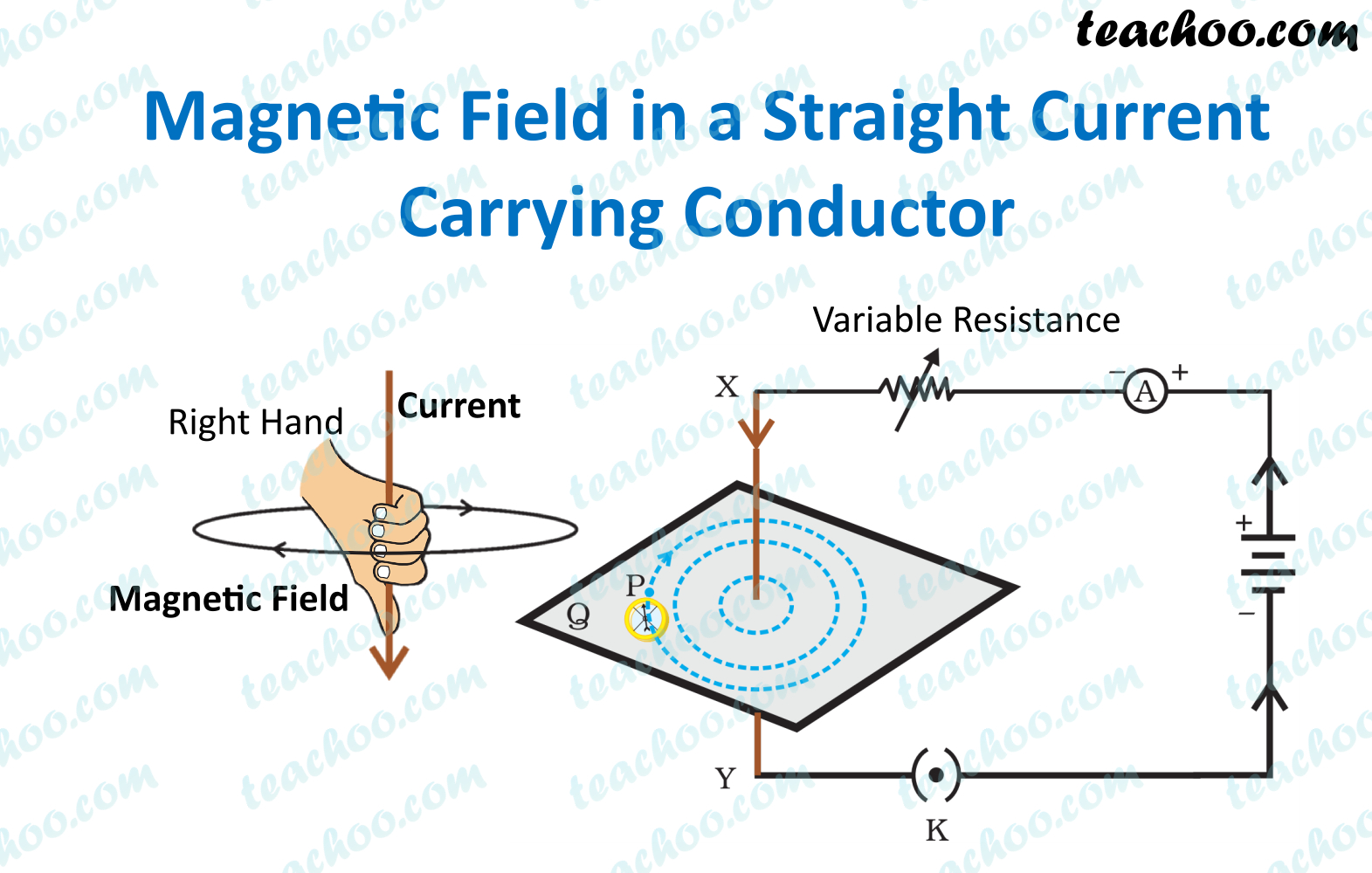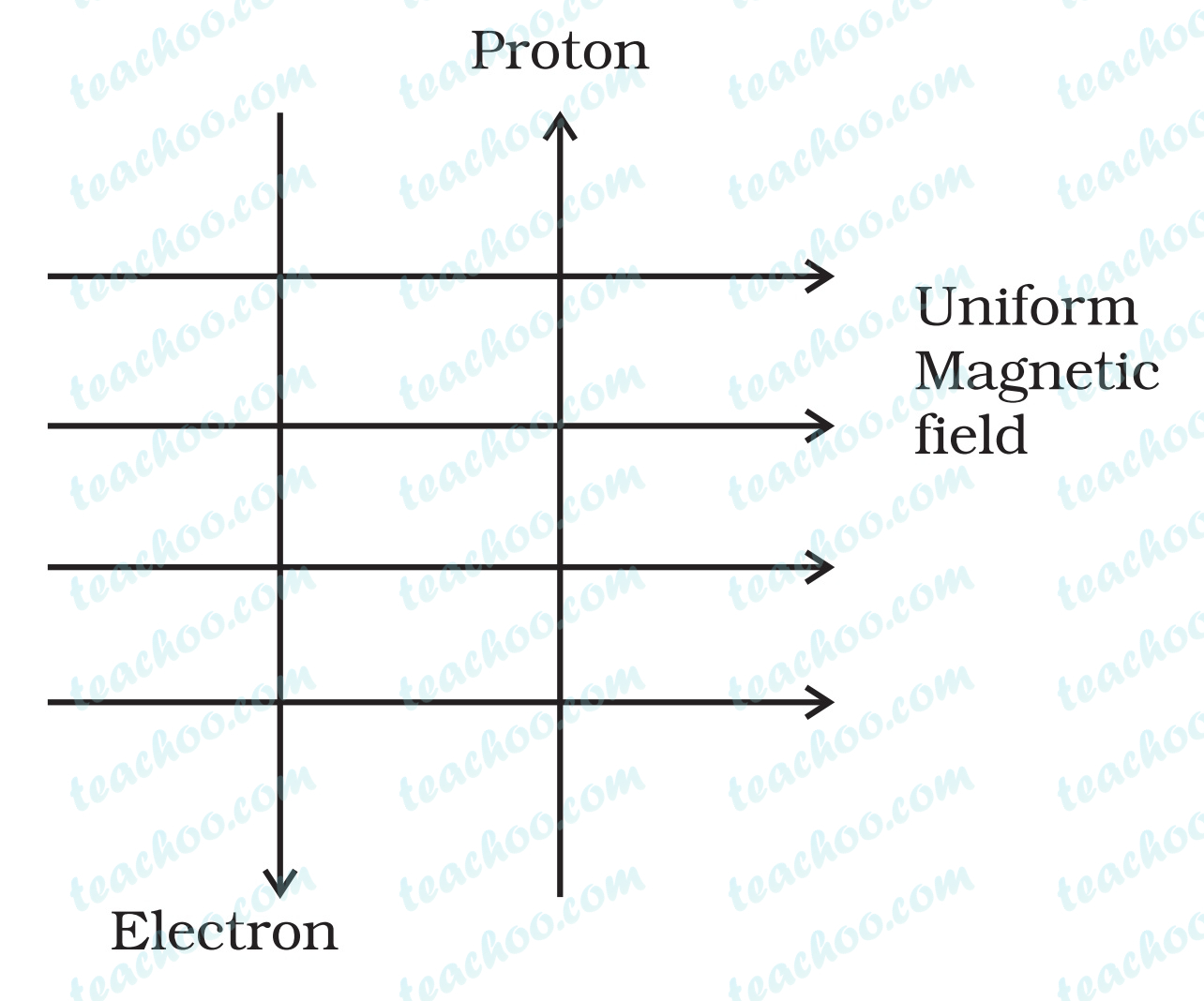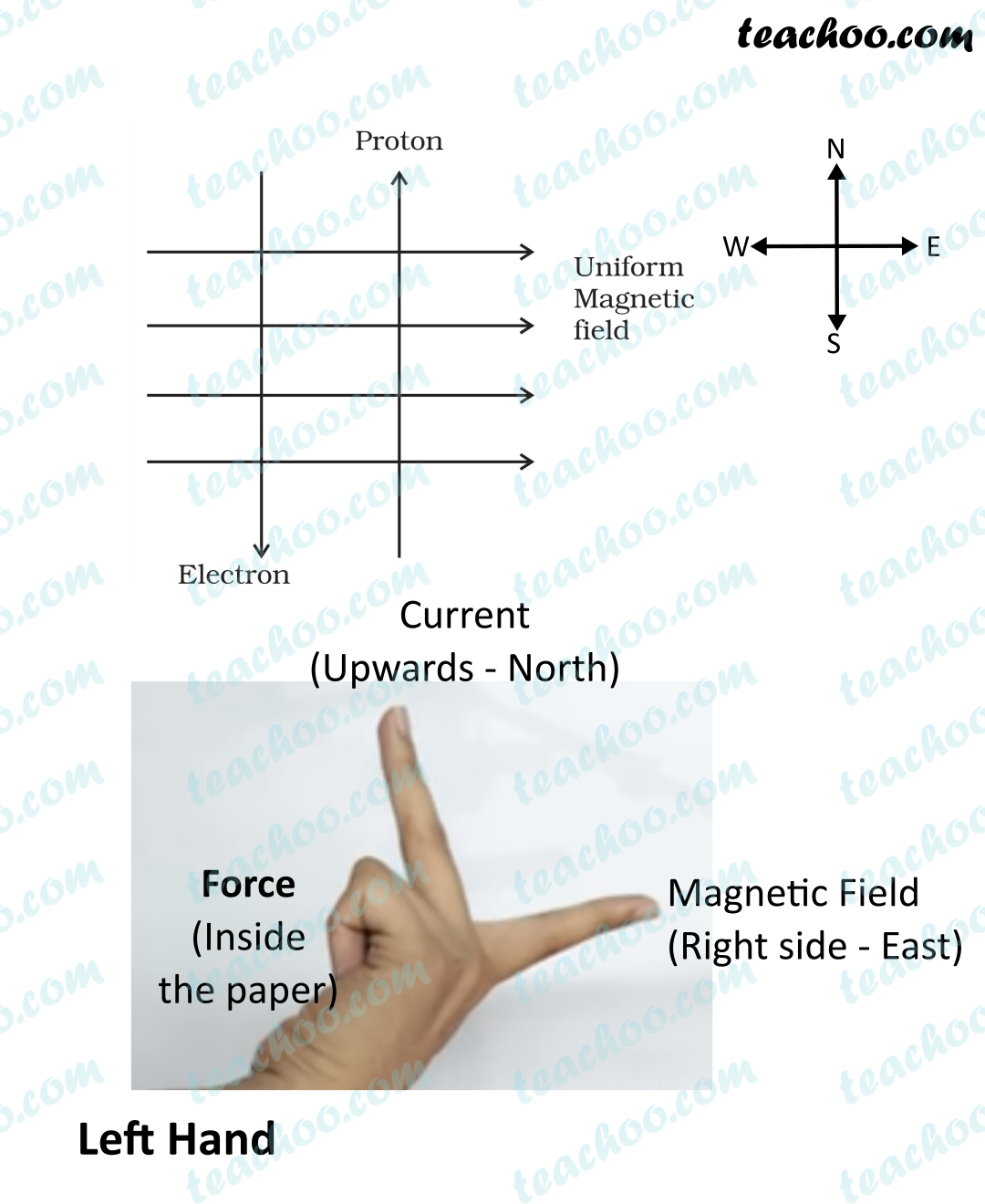Teachoo Questions

Class 10
Chapter 13 Class 10 - Magnetic Effects of Electric Current

The password to open the file is -    teachooisbest

## Choose the incorrect statement from the following regarding magnetic lines of field

(a) The direction of magnetic field at a point is taken to be the direction in which the north pole of a magnetic compass needle points

(b) Magnetic field lines are closed curves

(c) If magnetic field lines are parallel and equidistant, they represent zero field strength

(d) Relative strength of magnetic field is shown by the degree of closeness of the field lines

Here, (a), (b) and (d) are correct.

The incorrect statement is (c) If magnetic field lines are parallel and equidistant, they represent zero field strength

Explanation

If the magnetic field lines are parallel and equidistant, it represents uniform (same at all points) magnetic field.

### If the key in the arrangement (Figure 13.1) is taken out (the circuit is made open) and magnetic field lines are drawn over the horizontal plane ABCD, the lines are(a) concentric circles

(b) elliptical in shape

(c) straight lines parallel to each other

(d) concentric circles near the point O but of elliptical shapes as we go away from it

concentric circles

ExplanationThe conductor in the given figure is a straight current carrying wire

The magnetic field lines produced by a straight current carrying conductors are in the form of concentric circles.

### A uniform magnetic field exists in the plane of paper pointing from left to right as shown in Figure 13.3. In the field of an electron and a proton move as shown. The electron and the proton experience(a) forces both pointing into the plane of paper

(b) forces both pointing out of the plane of paper

(c) forces pointing into the plane of paper and out of the plane of paper, respectively

(d) force pointing opposite and along the direction of the uniform magnetic field respectively

forces both pointing into the plane of paper

Explanation

For Proton and Electron

 Proton Electron When proton moves upwards,  i.e. Positively charged particle moves upwards So, Direction of current is upwards When electron moves downwards,  i.e. Negatively charged particle moves downwards So, Direction of current is upwards

So, for both Proton and Electron,

Direction of Current i s upwards i.e. from South to North

And, Magnetic field is from West to East (Left to Right )Applying Fleming’s left hand rule,

Taking the forefinger, middle finger and thumb of the left hand in mutually perpendicular direction

• Pointing middle finger towards north direction (direction of current)
• Fore-finger towards east/right direction (direction of magnetic field)
• We get the direction of force is into the plane , since the thumb points into the plane.

So, direction of force for both electron and proton is

Into the plane

## Choose the incorrect statement

(a) Fleming’s right-hand rule is a simple rule to know the direction of induced current

(b) The right-hand thumb rule is used to find the direction of magnetic fields due to current carrying conductors

(c) The difference between the direct and alternating currents is that the direct current always flows in one direction, whereas the alternating current reverses its direction periodically

(d) In India, the AC changes direction after every 1/50 second

Here, (d) is the incorrect statement

Explanation

In India,

Frequency of AC current is 50 Hz

That means that the AC changes direction after every 1/100 second, not after 1/50 seconds

## To convert an AC generator into DC generator

(a) split-ring type commutator must be used

(b) slip rings and brushes must be used

(c) a stronger magnetic field has to be used

(d) a rectangular wire loop has to be used

(a) split-ring type commutator must be used

Explanation

Split rings are used to reverse the direction of current after every half revolution.

If split ring commutator will be used in AC generator, then the direction of current will be reversed after every half revolution.

Hence, a direct current will be produced.

Learn in your speed, with individual attention - Teachoo Maths 1-on-1 Class